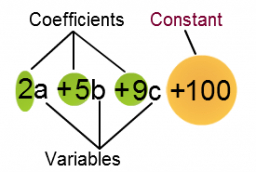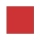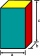# Simplify:

Simplify: 8(−9 − 5x) + 4b use x = 9 and b = −9

s =  -468

### Step-by-step explanation:Did you find an error or inaccuracy? Feel free to write us. Thank you!## Related math problems and questions:

• Evaluate 9Evaluate expression: 3 { 4 − 4 ( 5 − 12 ) } + 20
• Expression with bracketsEvaluate the expression. 15+[(9-4)×22]
• Simplify 2Simplify expression: 5ab-7+3ba-9
• When 5When 5 is subtracted from both my numerator and denominator I become 3/4. What fraction i am?
• SimplifySimplify powers multiplication: (3+22)(5-42)
• SimplifySimplify the following problem and express as a decimal: 5.68-[5-(2.69+5.65-3.89) /0.5]
• Evaluate expressionEvaluate following expression: 5 to the power of 2 - (4x4) divided by 2 =
• FloorRectangular floor of living room has a length 5.4 meters and a circumference 17.2 meters. What is its width?
• Similar trianglesThe triangles ABC and XYZ are similar. Find the missing lengths of the sides of the triangles. a) a = 5 cm b = 8 cm x = 7.5 cm z = 9 cm b) a = 9 cm c = 12 cm y = 10 cm z = 8 cm c) b = 4 cm c = 8 cm x = 4.5 cm z = 6 cm
• Find unknown 2Find unknown denominator: 2/3 -5/? = 1/4
• SelectSelect the statement that describes this expression: 5 x (3 + 4) - 4. a) 5 times the sum of 3 and 4, then subtract 4 b) 5 times 3 plus 4 minus 4 c) 5 more than the sum of 3 and 4, and then subtract 4 d) 5 times 3 plus the difference of 3 and 4 What is the
• Fractions and mixed numerals(a) Convert the following mixed numbers to improper fractions. i. 3 5/8 ii. 7 7/6 (b) Convert the following improper fraction to mixed number. i. 13/4 ii. 78/5 (c) Simplify these fractions to their lowest terms. i. 36/42 ii. 27/45 2. evaluate following ex
• Midpoint 5FM=3x-4, MG=5x-26, FG=? Point M is the midpoint of FG. Use the given information to find the missing measure or value.
• Edges of the cuboidFind the length of the edges of the cuboid, which has the following dimensions: width is 0.4 m; the height is 5.8 dm and the block can hold 81.2 liters of fluid.
• SimplifySimplify expression - which expression is equivalent to: 3(m + 2) − 4(2m − 9)
• Evaluate 12Evaluate and simplify 9 divided by 7/6.
• Distributive propertyVerify the distributive property a×(b+c)=(a×b)+(a*c) for the rational number a=5/8, b=7/4 and c=2/3